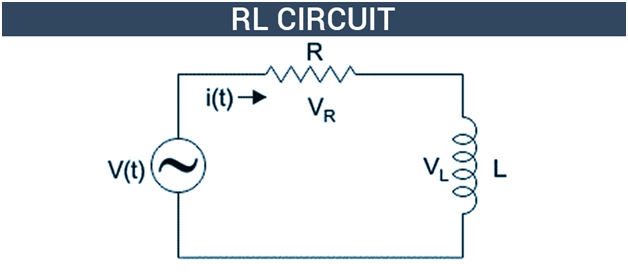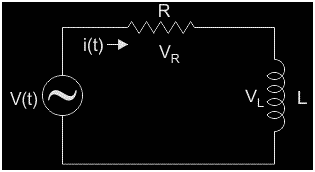# RL CircuitRL Circuits are those circuits which are purely the combination of inductors and resistors. An RL circuit has the inductor and a resistor connected in either parallel or series with each other, along with the current source operated by a voltage source.

As we already know, the process of induction occurs when an emf source is applied by a continuous change in the magnetic flux. The mutual inductance is an effect of the laws of induction of Faraday, while self-inductance is an effect of the laws of induction of Faraday of a device coming on itself. The inductor is a circuit or a device component which exhibits the self-inductance.

Consider the above circuit having a battery, a resistor, and a switch. The switch can either have the battery in the series connection to the circuit or the battery can be removed from the circuit. When the switch is closed, the current jumps to the maximum value and when the switch is opened, the value of current decreases immediately.

When an inductor is added in series with the resistor of the circuit, we come to observe changes in the current. The role of an inductor in the circuit is to oppose the change in the magnetic flux, i.e the inductor does not allow the spontaneous changes in the current. When we close the switch of the circuit, there is a gradual increase in the value of current to a maximum value. When we open the switch, removal of the battery, the inductor voltage causes the current to reduce gradually to the value of zero again.

## RL Series Circuit Equation

A circuit which contains a resistance R connected in series with the coil having an inductance L is known as an RL Series Circuit. When a supply voltage(V ) is applied across the current element I flowing in the circuit. IL and IR are the currents flowing in the inductor and resistor, but the current flowing across both the elements are the same as they are said to be connected in the series connection with each other. The diagram of the RL Series Circuit is as shown below-where VR is the voltage across resistor R

VL is the voltage across inductor L

V(t) is the total voltage across the circuit

In the above simple RL Series circuit where the resistor, R and the inductor, L are combined in series combination with the voltage source having V volts. The current flowing in the whole circuit is I amps and the current through the resistor and the inductor is IR and IL. Since both the resistance as well as the inductor are connected in the series combination, the current in both of the elements of the circuit remains to be the same.

Here, IL = IR = I. Consider VL and VR to be the voltage drops across the inductor and resistor. By the application of Kirchhoff voltage law (sum of the voltage drops must be the same across the circuit to apply the voltage) to this circuit, we get,

V(t) = VR + VL

Thus, this is the equation for the voltage across the RL series circuit.